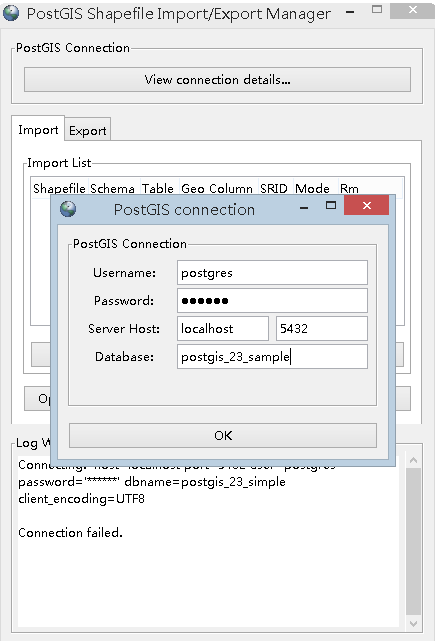## # 开源许可

• BSD许可：可以将修改后的代码作为开源或者专有软件再发布
• GPL许可：不允许修改后的代码做为闭源的商业软件发布和销售

## # 安装和使用

• 端口号默认：5432
• 超级管理员：postgres
• 在安装PostGIS前首先必须安装PostgreSQL，然后再安装好的Stack Builder中选择安装PostGIS组件
• 最好选择"Create spatial database"，以便在创建数据库时可以以此作为模板
• PostGIS安装目录需要和postgresql的安装目录一致
• 如果提示“找不到文件linintl-9.dll”，在postgresql/11/bin/postgisgui目录下，复制一份libintl-8.dll副本，改名为libintl-9.dll即可

#### # 远程访问（Windows）

• 修改配置文件/pgsql/data/pg_hba.conf，在IPV4部分添加新的一行
``````host all all 0.0.0.0/0 scram-sha-256
``````
• 重启服务：postgresql-64-14

#### # 远程访问（Linux）

• 修改配置文件postgresql.conf
``````vim /var/lib/pgsql/14/data/postgresql.conf

#port = 5432
port = 5432
``````
• 修改配置文件/pgsql/data/pg_hba.conf，同上
• 重启服务：postgresql-64-14

• 使用pgAdmin4管理数据库：如下，一个为二维数据库，一个为空间数据库• 使用PostGIS 2.0 Shapefile and DBF Loader Exporter 导入导出 .shp文件## # 数据库操作

#### # 模式（SCHEMA）

• 允许多个用户使用一个数据库并且不会互相干扰
• 将数据库对象组织成逻辑组以便更容易管理
``````-- 接下来我们连接到 runoobdb 来创建模式 myschema：
runoobdb=# create schema myschema;
-- 输出结果 "CREATE SCHEMA" 就代表模式创建成功

-- 接下来我们再创建一个表格
runoobdb=# create table myschema.company(
ID   INT              NOT NULL,
NAME VARCHAR (20)     NOT NULL,
AGE  INT              NOT NULL,
SALARY   DECIMAL (18, 2),
PRIMARY KEY (ID)
);
``````

#### # 三种自增列sequence，serial，identity区别

• sequence在所有数据库中的性质都一样，它是跟具体的字段不是强绑定的，其特点是支持多个对象之间共享，无特殊需求的情况下，作为最最次选
• identity本质是为了兼容标准sql中的语法而新加的，修复了一些serial的缺陷，比如无法通过alter table的方式实现增加或者删除serial字段，是serial的“增强版”，更适合作为“自增列”使用
• 共同的缺点是在显式插入之后，无法将自增值更新为表中的最大Id，一定要手动重置为表的最大Id

#### # 判断是否为 NULL

``````IF my_order_num IS NULL THEN
my_order_num = 1;
ELSE
my_order_num = my_order_num + 1;
END IF;
``````

#### # 拼接SQL语句

``````sql_query := 'SELECT column1, column2 FROM table_name WHERE ' || column_name;
``````

#### # SELECT 赋值

• 直接赋值到变量
``````-- 声明变量
DECLARE max_salary NUMERIC;

-- 将最大薪资赋给变量
SELECT MAX(salary) INTO max_salary FROM employees;
``````
• 将一个表中的列赋给一个记录变量
``````-- 声明记录变量
DECLARE max_value RECORD;

-- 使用 SELECT INTO 将结果集赋给变量
SELECT MAX(column_name) INTO max_value FROM table_name;

-- 使用
max_value.column_name
``````

#### # 获取当前时间

``````-- 带时区带毫秒
SELECT now();

-- 不带时区带毫秒
SELECT now()::timestamp;

-- 不带时区不带毫秒
SELECT now()::timestamp(0);
``````

#### # 游标的使用

• 需要先创建函数，将游标添加到函数中
``````CREATE OR REPLACE FUNCTION retrieve_employees() RETURNS VOID AS \$\$
DECLARE
emp_cursor CURSOR FOR SELECT name, salary FROM employees;
emp_name TEXT;
emp_salary NUMERIC;
BEGIN
OPEN emp_cursor;

LOOP
FETCH NEXT FROM emp_cursor INTO emp_name, emp_salary;

-- 处理数据
RAISE NOTICE 'Employee Name: %, Salary: %', emp_name, emp_salary;
END LOOP;

CLOSE emp_cursor;
END;
\$\$ LANGUAGE plpgsql;
``````
• 调用创建的函数
``````SELECT retrieve_employees();
``````

## # PostGIS中的几何类型

• PostGIS 是PostgreSQL的一个扩展，目的是使PostgreSQL支持空间数据的存储和使用，其本质类似于ArcSDE和Oracle Spatial Extension
• PostGIS支持所有OGC规范的Simple Features类型，同时还扩展了对3DZ、3DM、4D坐标的支持

### # OGC的WKB和WKT格式

OGC定义了两种描述几何对象的格式，分别是WKB（Well-Known Binary）和WKT（Well-Known Text）

``````//在SQL语句中，用以下的方式可以使用WKT格式定义几何对象：
POINT(0 0) //点
LINESTRING(0 0,1 1,1 2) //线
POLYGON((0 0,4 0,4 4,0 4,0 0),(1 1, 2 1, 2 2, 1 2,1 1)) //面
MULTIPOINT(0 0,1 2) //多点
MULTILINESTRING((0 0,1 1,1 2),(2 3,3 2,5 4)) //多线
MULTIPOLYGON(((0 0,4 0,4 4,0 4,0 0),(1 1,2 1,2 2,1 2,1 1)), ((-1 -1,-1 -2,-2 -2,-2 -1,-1 -1))) //多面
GEOMETRYCOLLECTION(POINT(2 3),LINESTRING((2 3,3 4))) //几何集合

//以下语句可以使用WKT格式插入一个点要素到一个表中
INSERT INTO table ( SHAPE, NAME )
VALUES ( GeomFromText('POINT(116.39 39.9)', 4326), '北京');
``````

### # EWKT、EWKB和Canonical格式

• EWKT和EWKB相比OGC WKT和WKB格式主要的扩展有3DZ、3DM、4D坐标和内嵌空间参考支持
• Canonical格式是16进制编码的几何对象，直接用SQL语句查询出来的就是这种格式
``````//以下以EWKT语句定义了一些几何对象：
POINT(0 0 0) //3D点
SRID=32632;POINT(0 0) //内嵌空间参考的点
POINTM(0 0 0) //带M值的点
POINT(0 0 0 0) //带M值的3D点
SRID=4326;MULTIPOINTM(0 0 0,1 2 1) //内嵌空间参考的带M值的多点

//以下语句可以使用EWKT格式插入一个点要素到一个表中
INSERT INTO table ( SHAPE, NAME )
VALUES ( GeomFromEWKT('SRID=4326;POINTM(116.39 39.9 10)'), '北京' )
``````

### # SQL-MM格式

SQL-MM格式定义了一些插值曲线，这些插值曲线和EWKT有点类似，也支持3DZ、3DM、4D坐标，但是不支持嵌入空间参考

``````//以下以SQL-MM语句定义了一些插值几何对象：
CIRCULARSTRING(0 0, 1 1, 1 0) //插值圆弧
COMPOUNDCURVE(CIRCULARSTRING(0 0, 1 1, 1 0),(1 0, 0 1)) //插值复合曲线
CURVEPOLYGON(CIRCULARSTRING(0 0, 4 0, 4 4, 0 4, 0 0),(1 1, 3 3, 3 1, 1 1)) //曲线多边形
MULTICURVE((0 0, 5 5),CIRCULARSTRING(4 0, 4 4, 8 4)) //多曲线
MULTISURFACE(CURVEPOLYGON(CIRCULARSTRING(0 0, 4 0, 4 4, 0 4, 0 0),(1 1, 3 3, 3 1, 1 1)),((10 10, 14 12, 11 10, 10 10),(11 11, 11.5 11, 11 11.5, 11 11))) //多曲面
``````

## # PostGIS空间信息处理

### # spatial_ref_sys表

``````//4326空间参考的srtext内容：
GEOGCS["WGS 84",DATUM["WGS_1984",SPHEROID["WGS 84",6378137,298.257223563,AUTHORITY["EPSG","7030"]],TOWGS84[0,0,0,0,0,0,0],AUTHORITY["EPSG","6326"]],PRIMEM["Greenwich",0,AUTHORITY["EPSG","8901"]],UNIT["degree",0.01745329251994328,AUTHORITY["EPSG","9122"]],AUTHORITY["EPSG","4326"]]

//4326空间参考的proj4text内容：
+proj=longlat +ellps=WGS84 +datum=WGS84 +no_defs
``````

### # geometry_columns表

geometry_columns表存放了当前数据库中所有几何字段的信息，比如我当前的库里面有两个空间表，在geometry_columns表中就可以找到这两个空间表中几何字段的定义：

### # 在PostGIS中创建一个空间表

``````//以下先在test模式下创建一个名为cities的一般表：
create table test.cities (id int4, name varchar(20))

//再给cities添加一个名为shape的几何字段（二维点）：
select AddGeometryColumn('test', 'cities', 'shape', 4326, 'POINT', 2)
``````

### # PostGIS对几何信息的检查

PostGIS可以检查几何信息的正确性，这主要是通过IsValid函数实现的

``````select IsValid('LINESTRING(0 0, 1 1)'), IsValid('LINESTRING(0 0,0 0)')
``````

``````ALTER TABLE cities
CHECK (IsValid(shape))
``````

``````INSERT INTO test.cities ( shape, name )
VALUES ( GeomFromText('LINESTRING(0 0,0 0)', 4326), '北京');

ERROR: new row for relation "cities" violates check constraint "geometry_valid"
SQL 状态: 23514
``````

### # PostGIS中的空间索引

``````CREATE INDEX shape_index_cities
ON sde.cities
USING gist
(shape);
``````

## # PostGIS中的常用函数

### # OGC标准函数

#### # 管理函数

``````添加几何字段 AddGeometryColumn(, , , , , )

``````

#### # 几何对象关系函数

``````获取两个几何对象间的距离 ST_Distance(geometry, geometry)

（比如LINESTRING(0 0, 2 2)和LINESTRING(0 0, 1 1, 2 2)是相同的几何对象） ST_Equals(geometry, geometry)

``````

#### # 几何对象处理函数

``````获取几何对象的中心 ST_Centroid(geometry)

``````

#### # 几何对象存取函数

``````获取几何对象的WKT描述 ST_AsText(geometry)

``````

#### # 几何对象构造函数

``````Text：WKT
WKB：WKB
Geom:Geometry
M:Multi
Bd:BuildArea
Coll:Collection ST_GeomFromText(text,[])

ST_PointFromText(text,[])
ST_LineFromText(text,[])
ST_LinestringFromText(text,[])
ST_PolyFromText(text,[])
ST_PolygonFromText(text,[])
ST_MPointFromText(text,[])
ST_MLineFromText(text,[])
ST_MPolyFromText(text,[])
ST_GeomCollFromText(text,[])
ST_GeomFromWKB(bytea,[])
ST_GeometryFromWKB(bytea,[])
ST_PointFromWKB(bytea,[])
ST_LineFromWKB(bytea,[])
ST_LinestringFromWKB(bytea,[])
ST_PolyFromWKB(bytea,[])
ST_PolygonFromWKB(bytea,[])
ST_MPointFromWKB(bytea,[])
ST_MLineFromWKB(bytea,[])
ST_MPolyFromWKB(bytea,[])
ST_GeomCollFromWKB(bytea,[])
ST_BdPolyFromText(text WKT, integer SRID)
ST_BdMPolyFromText(text WKT, integer SRID)
``````

### # PostGIS扩展函数

#### # 管理函数

``````删除一个空间表（包括geometry_columns中的记录） DropGeometryTable([], )

Geos：GEOS库
Jts：JTS库
Proj：PROJ4库 postgis_version()

postgis_lib_version()
postgis_lib_build_date()
postgis_script_build_date()
postgis_scripts_installed()
postgis_scripts_released()
postgis_geos_version()
postgis_jts_version()
postgis_proj_version()
postgis_uses_stats()
postgis_full_version()
``````

#### # 几何操作符

``````A范围=B范围 A = B
A范围覆盖B范围或A范围在B范围左侧 A &<> B
A范围在B范围左侧 A <<>> B
A范围覆盖B范围或A范围在B范围下方 A &<| B A范围覆盖B范围或A范围在B范围上方 A |&> B
A范围在B范围下方 A <<| B A范围在B范围上方 A |>> B
A=B A ~= B
A范围被B范围包含 A @ B
A范围包含B范围 A ~ B
A范围覆盖B范围 A && B
``````

#### # 几何量测函数

``````量测面积 ST_Area(geometry)

ST_length3d_spheroid(geometry,spheroid)

ST_perimeter2d(geometry)

``````

#### # 几何对象输出

``````参考语义：
NDR：Little Endian
XDR：big-endian
HEXEWKB：Canonical
SVG：SVG 格式
GML：GML 格式
KML：KML 格式
GeoJson：GeoJson 格式

ST_AsBinary(geometry,{'NDR'|'XDR'})
ST_AsEWKT(geometry)
ST_AsEWKB(geometry, {'NDR'|'XDR'})
ST_AsHEXEWKB(geometry, {'NDR'|'XDR'})
ST_AsSVG(geometry, [rel], [precision])
ST_AsGML([version], geometry, [precision])
ST_AsGeoJson([version], geometry, [precision], [options])
``````

#### # 几何对象创建

``````参考语义：
Dump：转储 ST_GeomFromEWKT(text)

ST_GeomFromEWKB(bytea)
ST_MakePoint(, , [], [])
ST_MakePointM(, , )
ST_MakeBox2D(, )
ST_MakeBox3D(, )
ST_MakeLine(geometry set)
ST_MakeLine(geometry, geometry)
ST_LineFromMultiPoint(multipoint)
ST_MakePolygon(linestring, [linestring[]])
ST_BuildArea(geometry)
ST_Polygonize(geometry set)
ST_Collect(geometry set)
ST_Collect(geometry, geometry)
ST_Dump(geometry)
ST_DumpRings(geometry)
``````

#### # 几何对象编辑

``````给几何对象添加一个边界，会使查询速度加快 ST_AddBBOX(geometry)

ST_RemovePoint(linestring, offset)
ST_SetPoint(linestring, N, point)

ST_Force_2d(geometry)
ST_Force_3dz(geometry), ST_Force_3d(geometry),
ST_Force_3dm(geometry)
ST_Force_4d(geometry)
ST_Multi(geometry)

ST_RotateX(geometry, float8)
ST_RotateY(geometry, float8)

ST_SimplifyPreserveTopology(geometry, tolerance)

ST_SnapToGrid(geometry, sizeX, sizeY), ST_SnapToGrid(geometry, size)

``````

#### # 线性参考

``````根据location（0-1）获得该位置的点 ST_line_interpolate_point(linestring, location)

ST_box3d(geometry)

0=2d, 1=3dm, 2=3dz, 3=4d ST_zmflag(geometry)

ST_npoints(geometry)

ST_YMin(box3d)
ST_ZMin(box3d)
ST_XMax(box3d)
ST_YMax(box3d)
ST_ZMax(box3d)

``````

#### # 长事务支持

``````启用/关闭长事务支持，重复调用无副作用 EnableLongTransactions()
DisableLongTransactions()

, )

, , , [])

``````